• python画函数图
千次阅读
2019-02-05 14:16:03
import numpy as np
import matplotlib.pyplot as plt

import warnings
warnings.filterwarnings('ignore')
#指数函数
#f(x) = a**x
x = np.linspace(-2,2,100)
y1 = (1/2)**x
y2 = (1/4)**x
plt.scatter(0,1,marker='.',color='k')
plt.text(0.01,1,r'(0,1)',fontsize=10)
plt.plot(x,y1,color='blue',linestyle='-')
plt.plot(x,y2,color='green',linestyle='-')
plt.axvline(0,color='gray',linestyle='--',alpha=0.8)
plt.axhline(0,color='gray',linestyle='--',alpha=0.8)
plt.axhline(1,color='red',linestyle='--',alpha=0.8)
plt.text(-2,4,r'(1/2)^x',fontsize=15)
plt.text(-2,16,r'(1/4)^x',fontsize=15)
plt.title('0<a<1')

plt.show()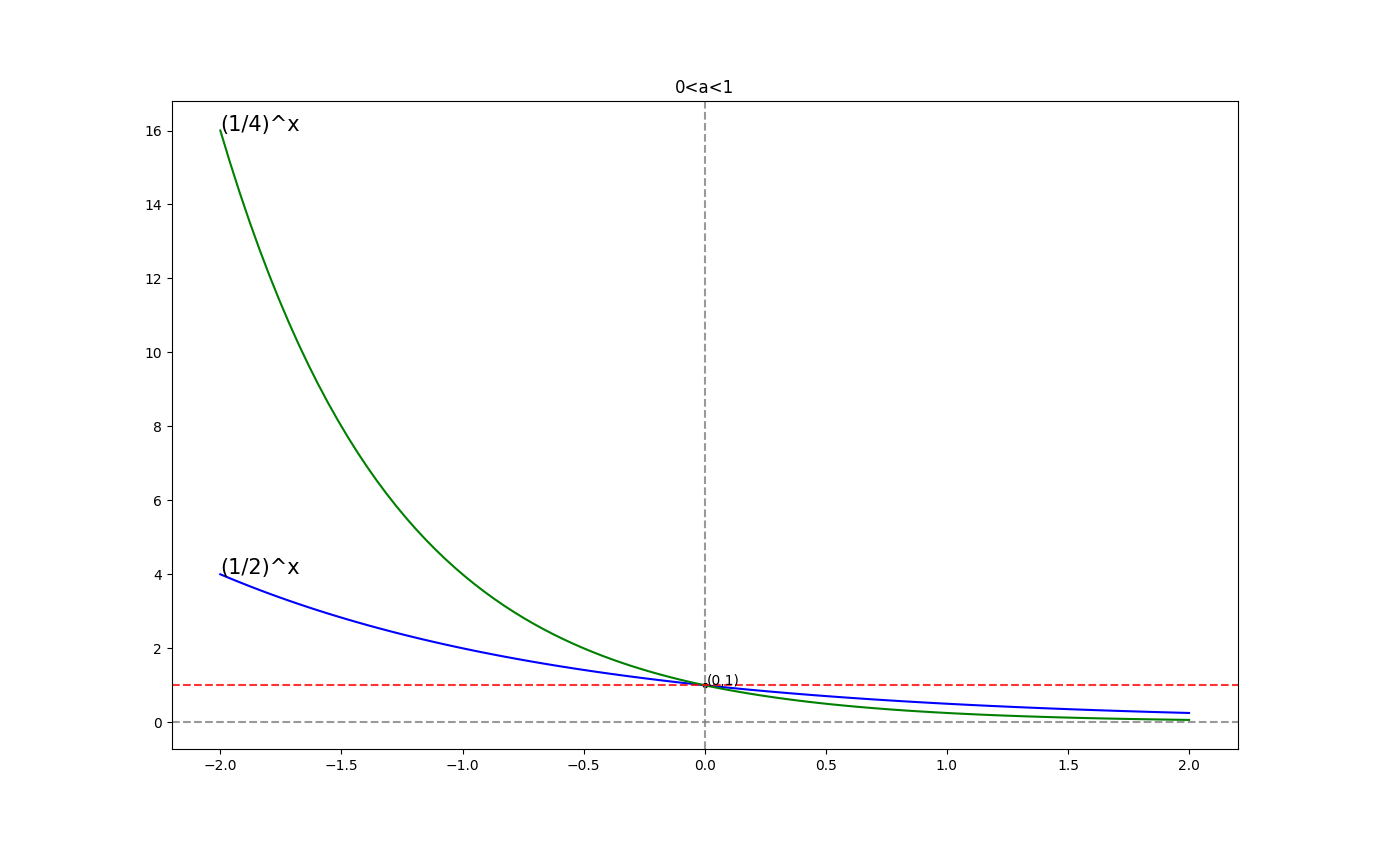更多相关内容
• ## 用python画函数图像

千次阅读 2020-12-18 22:40:41
sigmoid激活函数： import numpy as np import matplotlib.pylab as plt def sigmoid(x): return 1/(1+np.exp(-x)) # sigmoid函数 x = np.arange(-6.0,6.0,0.1) # 限定x的范围，给什么区间出来的就是在哪个...

画sigmoid激活函数：

import numpy as np
import matplotlib.pylab as plt

def sigmoid(x):
return 1/(1+np.exp(-x))  # sigmoid函数

x = np.arange(-6.0,6.0,0.1)  # 限定x的范围，给什么区间画出来的就是在哪个区间
y = sigmoid(x)  # 求y值

plt.plot(x,y)
plt.title("sigmoid")
plt.ylim(0,1) # 指定y轴的范围，画出来的效果不一样
plt.show()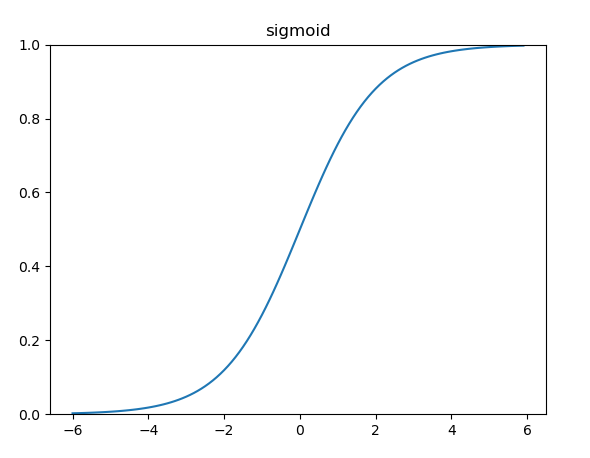画Relu激活函数：

import numpy as np
import matplotlib.pylab as plt

def relu(x):
return np.maximum(0,x)  # relu函数

x = np.arange(-6.0,6.0,0.1)  # 限定x的范围，给什么区间画出来的就是在哪个区间
y = relu(x)  # 求y值

plt.plot(x,y)
plt.title("relu")
plt.ylim(0,10) # 指定y轴的范围，画出来的效果不一样
plt.show()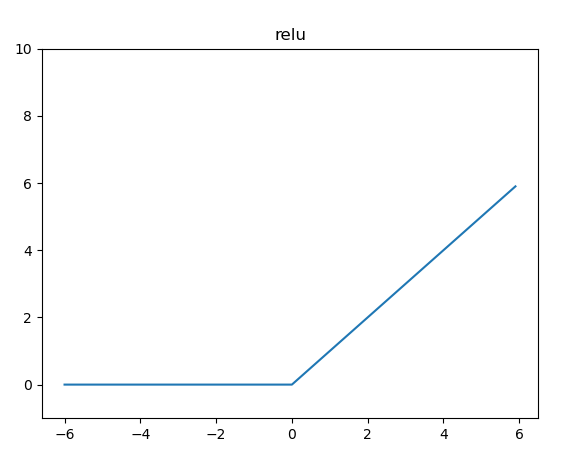画LeakyRelu激活函数

import numpy as np
import matplotlib.pylab as plt

def leakyrelu(x):
alpha = 0.1  # alpha的值并非固定
return np.maximum(alpha*x,x)

x = np.arange(-6.0,6.0,0.1)  # 限定x的范围，给什么区间画出来的就是在哪个区间
y = leakyrelu(x)  # 求y值

plt.plot(x,y)
plt.title("leakyrelu")
plt.ylim(-2,5) # 指定y轴的范围，画出来的效果不一样
plt.show()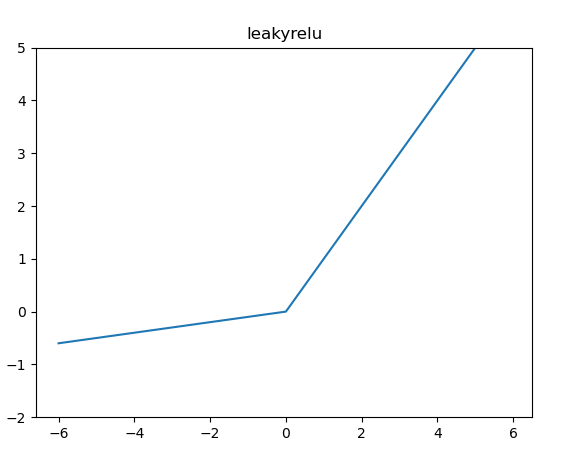画一元二次函数：

import numpy as np
import matplotlib.pylab as plt

def func(x):
return (x**2+2*x+1)  # 一元二次函数

x = np.arange(-6.0,6.0,0.1)  # 限定x的范围，给什么区间画出来的就是在哪个区间
y = func(x)  # 求y值

plt.plot(x,y)
plt.title("f(x)=x**2+2x+1")
plt.ylim(0,10) # 指定y轴的范围，画出来的效果不一样
plt.show()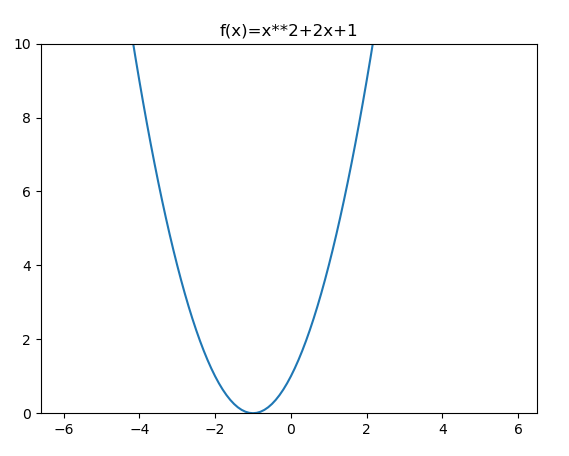展开全文开发语言 后端
• 因为这个函数的范围太大, 并且在三分之一处出现断崖分布, 必须得缩小x轴和y轴的范围, 不然画图的误差会相当大: lt.xlim((-0.6, 0.6)) plt.ylim((-0.6, 0.6)) 5. 对图像进行些许的美化: ax = plt.gca() ax.spines['...

目的: 发出 y = x ** 3 / (x * 3 + 1) 的图像

1. 导入matplotlib模块还有numpy模块:

import numpy as np
import matplotlib.pyplot as plt

2. 设置一个x的大致范围, 和y相对应的值域:

x = np.arange(-100, 100, 0.001)
y = x ** 3 / (3 * x + 1)


3. 使用figure创建画布, 把参数传进plot里面:

plt.figure()
plt.plot(x, y, linestyle='--', color='red')

4. 因为这个函数的范围太大, 并且在三分之一处出现断崖分布, 必须得缩小x轴和y轴的范围, 不然画图的误差会相当大:

lt.xlim((-0.6, 0.6))
plt.ylim((-0.6, 0.6))

5. 对图像进行些许的美化:

ax = plt.gca()
ax.spines['right'].set_color('none')
ax.spines['top'].set_color('none')
ax.xaxis.set_ticks_position('bottom')
ax.yaxis.set_ticks_position('left')
ax.spines['bottom'].set_position(('data', 0))
ax.spines['left'].set_position(('data', 0))

6. 最后要使用show展示出来:

plt.show()

7. 效果如下: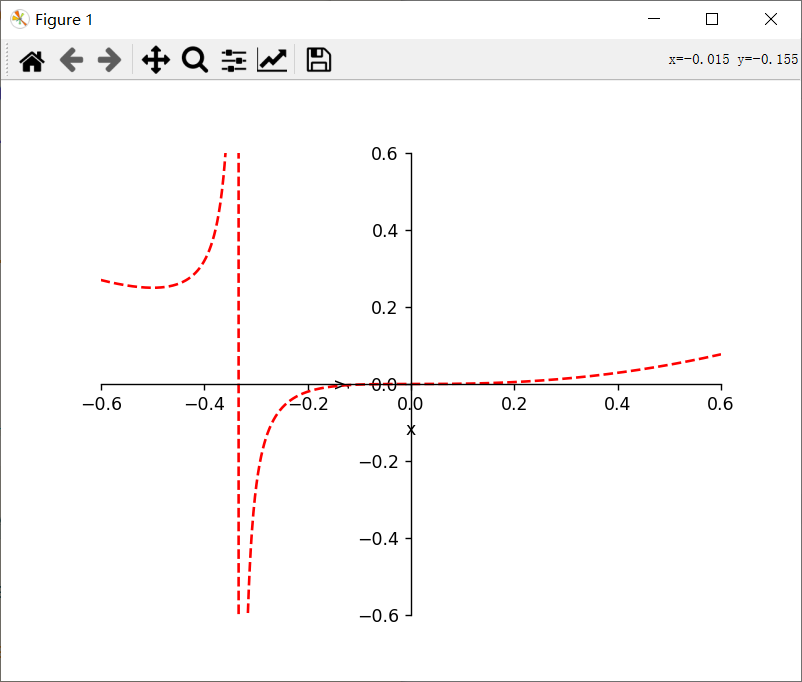8. 所用代码:

import numpy as np
import matplotlib.pyplot as plt

x = np.arange(-100, 100, 0.001)
y = x ** 3 / (3 * x + 1)

plt.figure()
plt.plot(x, y, linestyle='--', color='red')
plt.xlim((-0.6, 0.6))
plt.ylim((-0.6, 0.6))
plt.xlabel('x')
plt.ylabel('y')
ax = plt.gca()
ax.spines['right'].set_color('none')
ax.spines['top'].set_color('none')
ax.xaxis.set_ticks_position('bottom')
ax.yaxis.set_ticks_position('left')
ax.spines['bottom'].set_position(('data', 0))
ax.spines['left'].set_position(('data', 0))
plt.show()


展开全文• 下面我们尝试用Python的2D绘图库matplotlib来绘制函数图像。下面我们来实现一个简单的函数：首先，调用matplotlib库和numpy库import matplotlib.pyplot as pyplotimport numpy然后，确定绘制图像的定义域范围（例如...通过图像可以直观地学习函数变化、分布等规律，在学习函数、概率分布等方面效果显著。下面我们尝试用Python的2D绘图库matplotlib来绘制函数图像。

下面我们来实现一个简单的函数：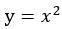首先，调用matplotlib库和numpy库import matplotlib.pyplot as pyplot

import numpy

然后，确定绘制图像的定义域范围（例如我们使用[-10,10]区间），并使用numpy的linspace方法在目标范围内等距地选取200个自变量。在这里选取的点数越多，函数绘制得会越精细。x=numpy.linspace(-10, 10, 200)

接着，计算所有自变量对应的因变量。y=[i**2 for i in x]

最后，我们用pyplot绘制图像并显示它。当使用plot方法绘制函数图像时，pyplot会自动将所有相邻的点之间用直线连接。pyplot.plot(x,y)

pyplot.show()

此时的绘图结果如下：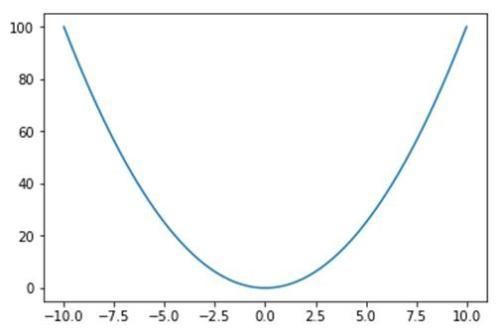完整代码如下：import matplotlib.pyplot as pyplot

import numpy

x=numpy.linspace(-10,10,200)

y=[i**2 for i in x]

pyplot.plot(x,y)

pyplot.show()

展开全文• python绘制函数图像代码，NumPy(Numerical Python) 是 Python 语言的一个扩展程序库，支持大量的维度数组与矩阵运算，此外也针对数组运算提供大量的数学函数库。 NumPy 的前身 Numeric 最早是由 Jim Hugunin 与其它...
• Python如何画函数的曲线输入以下代码导入小编们用到的函数库。 >>> import numpy as np >>> import matplotlib.pyplot as plt >>> x=np.arange(0,5,0.1); >>> y=np.sin(x); plt.plot(x,y) 采用刚才代码后有可能无法...
• 例1：函数 的图像 import matplotlib.pyplot as plt import numpy as np plt.figure() x = np.arange(-3.5,7.5,0.01) y = x**3-5*x**2-4*x+20 plt.plot(x,y) plt.show() 例2： 函数的图像 ...pyplot matplotlib
• ## Python绘制函数图像

万次阅读 多人点赞 2020-06-03 21:44:09
一元一次函数 一元二次函数 指数函数 正余弦函数 二元一次函数 二元二次函数 导入相关包 from mpl_toolkits.mplot3d import Axes3D import numpy as np from matplotlib import pyplot as plt plt.rcParams...
• 废话少说，直接上代码： ... # 这里的函数可以任意定义 return np.sum(x**2) fig = plt.figure() ax = Axes3D(fig) x = np.arange(-3,-3,0.1) y = np.arange(-3,-3,0.1) X,Y = np.meshgrid(x,y)#创建网格，这个是关键
• python实现绘制二次函数图像 python实现绘制三维函数图像 python实现绘制二次函数图像 import matplotlib.pyplot as plt import numpy as np import math x = np.linspace(-10, 10, 10) #分别代表最小，最大，...开发语言 后端
• Python 非常热门，但除非工作需要没有刻意去了解更多，直到有个函数图要绘制，想起了它。结果发现，完全用不着明白什么是编程，就可以使用它完成很多数学函数图的绘制。通过以下两个步骤，就可以进行数学函数的绘制...
• Python 非常热门，但除非工作需要没有刻意去了解更多，直到有个函数图要绘制，想起了它。结果发现，完全用不着明白什么是编程，就可以使用它完成很多数学函数图的绘制。通过以下两个步骤，就可以进行数学函数的绘制...
• ## 用Python绘制数学函数图像

万次阅读 多人点赞 2021-05-26 16:13:23
博客文章: ...答案是有很多的，有很多不错的软件都能函数图像，但是，我想到了Python的数据可视化。Python在近些年非常火热，在数据分析以及深度学习等方面得到广泛地运用，其丰富的库使其功能愈.numpy
• 2.3.2例子二次函数绘制方法把二次函数图像变成点存储于list中直线的时候我们了三个点，我们还可以更多的点。点越多图像就越是一条直线，运用的思想是微积分的思想。如何生成很多点呢?python本身自带range()...
• 这里用Python逼近函数y = exp(x);同样使用泰勒函数去逼近:exp(x) = 1 + x + (x)^2/(2!) + .. + (x)^n/(n!) + ...#!/usr/bin/python# -*- coding:utf-8 -*-import numpy as npimport mathimport matplotlib as mpl...
• 需要的库：matplotlib 用法： importmatplotlib.pyplot as plt plt.plot(x, y) #x...np.linspace(-10, 10, 1000) y= [math.sin(t) for t inx] plt.plot(x, y) plt.show() 效果： 我们可以改变y的取值生成各种函数图像。
• 主要介绍了python 图像处理一个正弦函数代码实例,文中通过示例代码介绍的非常详细，对大家的学习或者工作具有一定的参考学习价值,需要的朋友可以参考下
• ## 用python画三角函数

千次阅读 2020-12-04 19:52:12
Pyplothttp://www.labri.fr/perso/nrougier/teaching/matplotlib/pyplot提供了一个方便的matplotlib...简单的画图simple plot我们想在同一个图中划出一个cos和sin函数图，第一步是获取cos和sin的数据，构建函数imp...
• 这里用Python逼近函数y = exp(x);同样使用泰勒函数去逼近: exp(x) = 1 + x + (x)^2/(2!) + .. + (x)^n/(n!) + … #!/usr/bin/python # -*- coding:utf-8 -*- import numpy as np import math import matplotlib as ...
• 主要介绍了Python绘制正余弦函数图像的方法，小编觉得挺不错的，现在分享给大家，也给大家做个参考。一起跟随小编过来看看吧
• python绘制一个简单的函数图像（B站视频） 实现的思想 其实就是我们先把点给设置好，然后就可以描点连线了 就是使用的matplotlib这个包下面的pylib这个模块下面的 plot（）和scantter（）方法来实现的 这两个包的...java javascript
•机器学习 数据挖掘
• python中有很多功能强大的模块，这篇经验告诉你，如何利用python的matplotlib模块，绘制正弦函数y=sin(x)的图像。工具/原料windows系统电脑一台python软件，并安装由matplotlib模块和numpy模块方法/步骤1第一步，...
• (x^2+9/4*y^2+z^2-1)^3-x^2*z^3-9/80*y^2*z^3==0
• 这篇经验告诉你，如何利用python的matplotlib模块三角函数的图像，包括正弦、余弦、正切、余切。工具/原料windows系统电脑一台python软件，并安装matplotlib模块、numpy模块spyder编辑器方法/步骤1第一步，点击键盘 ...
• 导入必要的库 import math import matplotlib.pyplot as plt import numpy as np import matplotlib as mpl ...mpl.rcParams['axes.unicode_minus...绘制softmax函数图像 fig = plt.figure(figsize=(6,4)) ax...
• python中有很多功能强大的模块，这篇经验告诉你，如何利用python的matplotlib模块，绘制指数函数的图像。工具/原料windows系统电脑一台python软件，并安装由matplotlib模块和numpy模块方法/步骤1第一步，点击键盘 ...
• ## python画分段函数

千次阅读 2022-04-11 15:43:49
import matplotlib.pyplot as plt import numpy as np x = np.linspace(-10, 10, 100) y = [i if (i>0) else 0 for i in x] plt.figure() plt.plot(x, y) plt.title("relu") plt.xlabel("np") ...
• 为了让用户能够使用python时，方便地绘制 2D 图表，PYTHON的模块中提供Matplotlib模块中所含的子库pyplot，提供了和 MATLAB 类似的绘图功能。Pyplot 包含一系列绘图函数的相关函数，每个函数会对当前的图像进行一些......